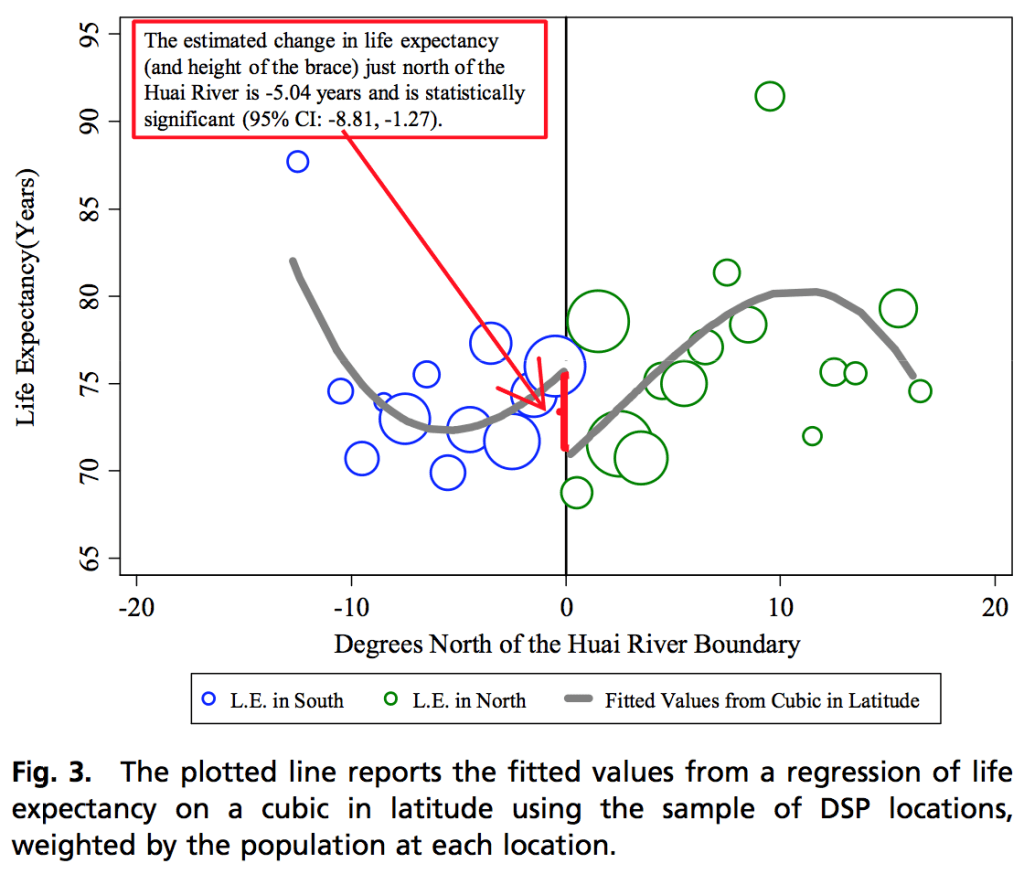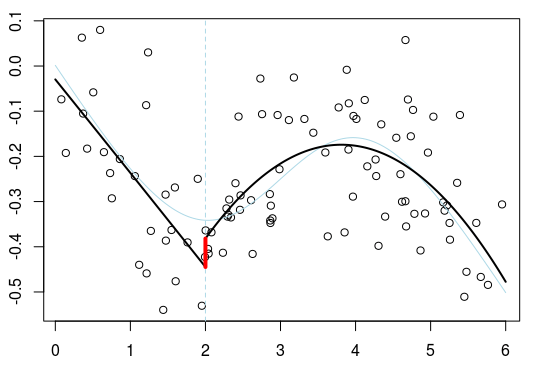[This article was first published on R-english – Freakonometrics, and kindly contributed to R-bloggers]. (You can report issue about the content on this page here)
Want to share your content on R-bloggers? click here if you have a blog, or here if you don't.

There are some graphs that you cannot forget. One graph that I found puzzling was mentioned on Andrew Gelman’s blog, a few years back, and was related to rupture detectionWhat I remember from this graph is that if you want to get a rupture, you can easily find one…

Recently, I had to review a paper, and Imbens & Lemieux (2008) was mentioned (the paper on Regression Discontinuity). It is a great reference and a major contribution, undoublty. But now, each time I read about ruptures and discontinuities, I have this graph in mind, and I start having doubt. I there really a discontinuity ? or is it somehow artificial, simply because the author is looking for a discontinuity ? For instance, consider the following (simulated) dataset

```f=function(x) pnorm(x,mean=3)-x/4 n=100 set.seed(1) X=runif(n)*6 Y=f(X)+rnorm(n)/8 DB=data.frame(X,Y)```It is a smooth continuous model. To test for discontinuity, a standard procedure seems to fit models on the left, and on the right. For instance two linear regression models,

```s=2 i=1 reg1=lm(Y~poly(X,i),data=DB,subset=X<=s) reg2=lm(Y~poly(X,i),daia=DB,subset=X>=s) u=seq(0,6,by=.025) v1=predict(reg1,newdata=data.frame(X=u[u<=s])) v2=predict(reg2,newdata=data.frame(X=u[u>=s])) lines(u[u<=s],v1,lwd=2) lines(u[u>=s],v2,lwd=2)```or two quadratic ones,

```i=2 reg1=lm(Y~poly(X,i),data=DB,subset=X<=s) reg2=lm(Y~poly(X,i),daia=DB,subset=X>=s) u=seq(0,6,by=.025) v1=predict(reg1,newdata=data.frame(X=u[u<=s])) v2=predict(reg2,newdata=data.frame(X=u[u>=s])) lines(u[u<=s],v1,lwd=2) lines(u[u>=s],v2,lwd=2)```We observe here a discontinuity… but is it significant ? Consider here simulations of the dataset, and try several breakpoints

```SIMU=function(ns=1e3){ MAT=matrix(NA,ns,21) n=100 for(j in 1:ns){ X=runif(n)*6 Y=f(X)+rnorm(n)/8 DB=data.frame(X,Y) saut=function(s){ reg1=lm(Y~poly(X,2),data=DB,subset=X<=s) reg2=lm(Y~poly(X,2),data=DB,subset=X>=s) predict(reg2,newdata=data.frame(X=s))-predict(reg1,newdata=data.frame(X=s)) } S=seq(.5,5.5,by=.25) MAT[j,]=Vectorize(saut)(S) } return(MAT)}```

If we plot those differences, between the model on the left and the model on the right of the breakpoint, we get

```SM=SIMU(1e3) S=seq(.5,5.5,by=.25) plot(S,SM[1,],type="l",ylim=c(-.7,.5),col="light blue") for(j in 2:100) lines(S,SM[j,],col="light blue") abline(h=0,lty=2) lines(S,apply(SM,2,mean),col="red",lwd=2) lines(S,apply(SM,2,function(x) quantile(x,.9)),col="red") lines(S,apply(SM,2,function(x) quantile(x,.1)),col="red")```for various breakpoints. The good thing is that it looks like we should reject the idea of having a breakpoint here, since it might not be “significant”. But what if the change of concavity was sharper, in our simulated dataset

```f=function(x) pnorm(x,mean=3)-x/4 n=100 set.seed(1) X=runif(n)*6 Y=f(X)+rnorm(n)/8 DB=data.frame(X,Y)```With a simular code, we might now accept the idea of having a discontinuity, that we do not have actually…But more puzzeling, if we get back to the initial statement in the post of Andrew Gelman, it might actually be possible to chose the degrees of the polynomial regressions to validate the discontinuity hypothesis. Let us get back on our original dataset.```SIMU=function(ns){ MAT=matrix(NA,ns,21) n=100 for(j in 1:ns){ X=runif(n)*6 Y=f(X)+rnorm(n)/8 DB=data.frame(X,Y) saut=function(s){ reg1=reg2=rep(NA,3) for(i in 1:3){ reg1[i]=predict(lm(Y~poly(X,i),data=DB,subset=X<=s),newdata=data.frame(X=s)) reg2[i]=predict(lm(Y~poly(X,i),data=DB,subset=X>=s),newdata=data.frame(X=s)) } max(abs(rep(reg1,each=3)-rep(reg2,3)))} S=seq(.5,5.5,by=.25) MAT[j,]=Vectorize(saut)(S) } return(MAT)}```

Here we try a linear regression, a quadratic and a cubic one. If we generate one thousand datasets, we get

```SM=SIMU(1e3) S=seq(.5,5.5,by=.25) plot(S,SM[1,],type="l",ylim=c(-.1,.9),col="light blue") for(j in 2:100) lines(S,SM[j,],col="light blue") abline(h=0,lty=2) lines(S,apply(SM,2,mean),col="red",lwd=2) lines(S,apply(SM,2,function(x) quantile(x,.9)),col="red") lines(S,apply(SM,2,function(x) quantile(x,.1)),col="red")```i.e. wherever we seek a discontinuity, we can actually find one. Even if we did generate a very smooth regression model….